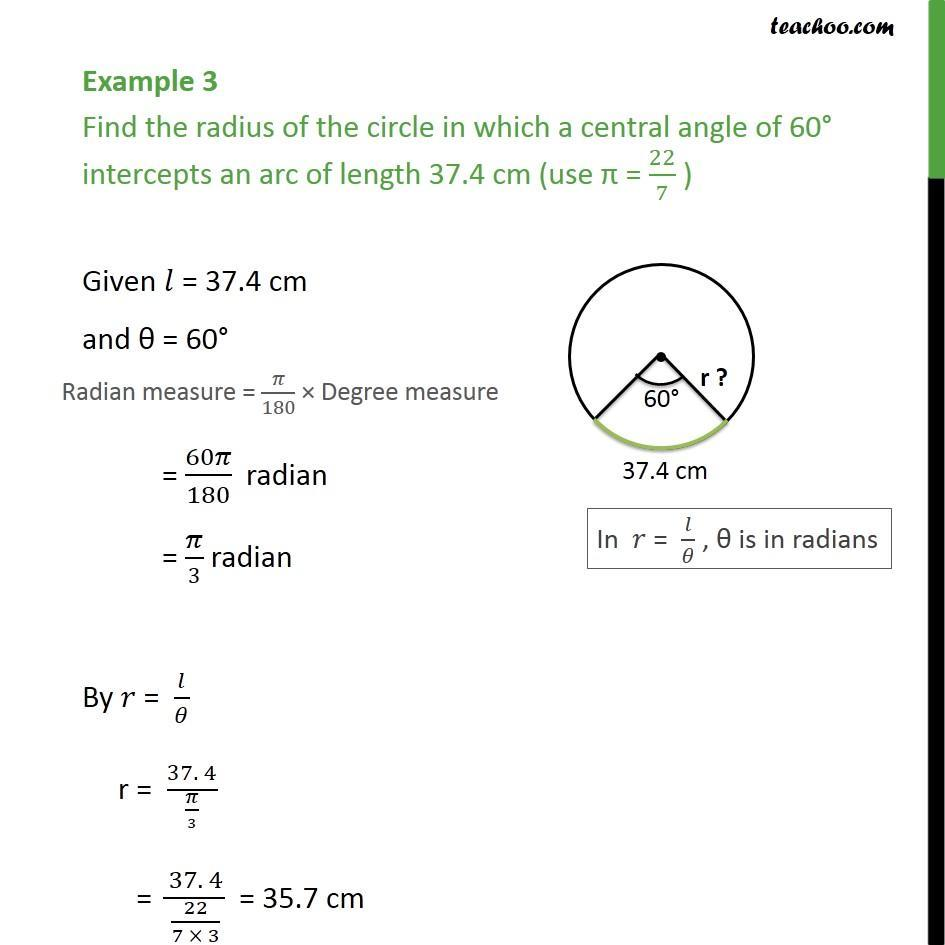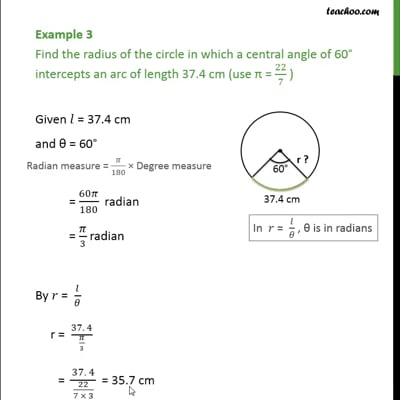Arc length

Chapter 3 Class 11 Trigonometric Functions
Concept wiseThis video is only available for Teachoo black users

Introducing your new favourite teacher - Teachoo Black, at only ₹83 per month

### Transcript

Example 3 Find the radius of the circle in which a central angle of 60 intercepts an arc of length 37.4 cm (use = 22/7 ) Given = 37.4 cm and = 60 = 60 /180 radian = /3 radian By = / r = (37. 4)/( /3) = (37. 4)/(22/(7 3)) = 35.7 cm